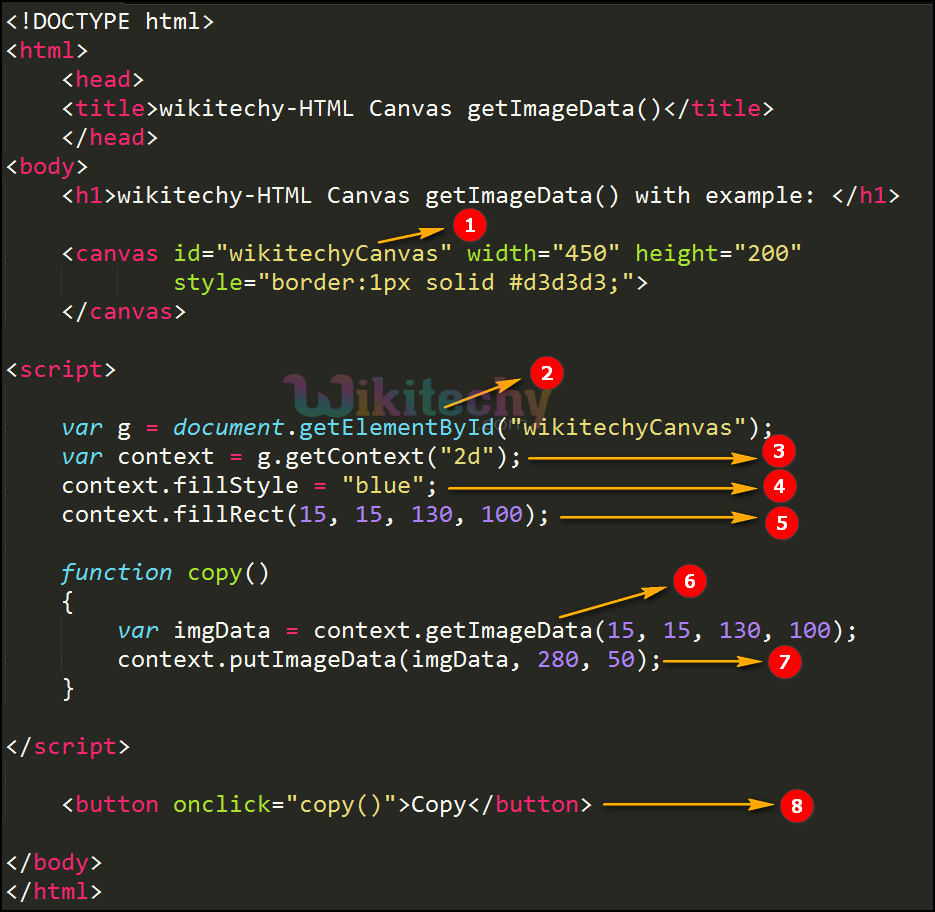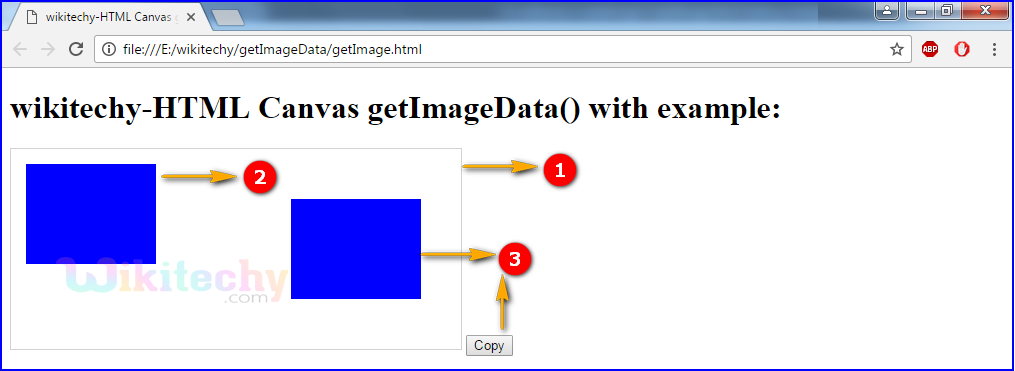# html tutorial - getImageData() Method in HTML5 Canvas - html5 - html code - html formLearn html - html tutorial - Getimagedata method in html5 canvas - html examples - html programs

• The getImageData() method is used to returns an ImageData object.
• The ImageData object copies the pixel data for the specified rectangle on a canvas.
• Every pixel in an imageData object has four pieces of information, there are RGBA value.
• R- The red color (from 0 to 255).
• G-The green color (from 0 to 255).
• B-The blue color (from 0 to 255).
• A-the alpha channel (from 0 to 255)
• Where 0 s transparent and 255 is fully visible.
• The alpha/ color information is held in array and is stored in the data property of the imageData object.

## Property values for getImageData() Method in HTML5 Canvas:

Parameter Description
x To start copy from upper left corner the x-coordinate in pixels.
y To start copy from upper left corner the y-coordinate in pixels
width Copy the width of rectangle
height Copy the height of rectangle

## Code Explanation for getImageData() method in HTML5 Canvas:1. “wikitechyCanvas” is used to declare the id value of the canvas tag.
2. getElementById() method is used to draw the element from id.
3. getContext(): returns an object that provides methods and properties for drawing on the canvas.
4. The fillStyle property is used to fill the blue color in rectangle.
5. To create a rectangle the value is set as (15, 15, 130, 100) in (x,y,width,height) axis.
6. The getImageData(15, 15, 130, 100) is used to copy the above rectangle.
7. The image will put back on the canvas using putImageData(imgData,280 ,50 ).
8. Click button is used for call the “Function copy() “ Method.

## Output for getImageData() Method in HTML5 Canvas:1. canvas is used to draw a rectangle an getImageData() method.
2. Here the output is displayed by blue color rectangle.
3. The first rectangle is displayed by (15, 15, 130, 100) in (x,y,width,height) axis.
4. The above rectangle copied by using the copy button that will be used for get the value of the imageData.

## Browser Support for getImageData() Method in HTML5 Canvas:Yes 9.0 Yes Yes Yes

## Tips and Notes

• The color/alpha information in the array is manipulated .
• The image data can copy on back to the canvas with the putImageData() method.
• The ImageData object is not a picture, it specifies a part (rectangle) on the canvas, and holds information of every pixel inside that rectangle.
• To use the getImageData() method invert the color of every pixels of an image on the canvas.